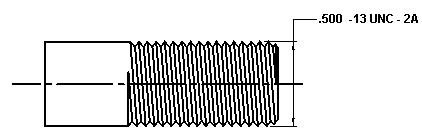Tensile Thread Stress Area Equation and Calculator - 100 ksi and More

(This calculator requires a java - enabled browser)

This calculator calculates the tensile stress area of a external screw thread of a fastener. Use this calculator for materials over 100,000 psi ultimate tensile strength.

This calculator will calculate the unknown for the given conditions. Two (2) inputs are required, See the application illustration at bottom of page.Where:

At = Tensile Strength Area of Screw Thread.
Esmin = MinimumPitch Diameter of External Thread - Thread Engineering Data Chart
n = Number of threads per inch

This equation is a more conservative extimation of actual thread stress area as opposed to the equation for Tensile Thread Stress Area 100 ksi and Less.Example; For a 1/2 - 13 UNC - 2A, see Thread Engineering Data Chart for minimum pitch diameter data.

Esmin = .4435
n = 13

At = 3.1416 (.4435/2 - 0.16238/13)2
At = .13757 = (Thread Stress Area)

Related:Membership Register | LoginHomeEngineering Book StoreEngineering ForumExcel App. DownloadsOnline Books & ManualsEngineering NewsEngineering VideosEngineering CalculatorsEngineering ToolboxGD&T Training Geometric Dimensioning TolerancingDFM DFA TrainingTraining Online EngineeringAdvertising CenterCopyright Notice